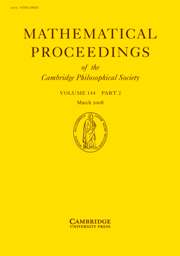# Morris' paper accepted to Math Proc Camb Phil SocIan Morris’ paper on “Extremal sequences of polynomial complexity“, joint with Nikita Sidorov (University of Manchester) and Kevin Hare (University of Waterloo, Canada), has recently been accepted for publication in the Mathematical Proceedings of the Cambridge Philosophical Society.  A summary of the paper follows.  The joint spectral radius of a finite set of square matrices is defined to be the largest possible exponential growth rate of long products of matrices drawn from that set. In the influential 1995 article “The finiteness conjecture for the generalized spectral radius of a set of matrices“, Jeffrey Lagarias and Yang Wang conjectured that this maximum possible rate of growth can always be realized by multiplying together a periodic sequence of matrices.  This conjecture was shown to be false in 2002 by Thierry Bousch and Jean Mairesse, who gave an example in which the maximum growth rate is only achieved by sequences of matrices which have at least linear subword complexity.  In this article the authors investigate how strongly Lagarias and Wang’s conjecture can fail to be true by constructing an example in which the maximum growth rate can only be achieved by sequences of matrices which have at least polynomial subword complexity.  The final form preprint is available on the arXiv.